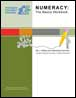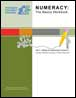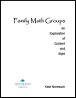# Subtraction

## Numeracy: The Basics Workbook – Set J: Adding & Subtracting Decimals (2012)This is one in a series of workbook intended to accompany Workplace Education Manitoba’s (WEM’s) Numeracy: The Basics video series. There are 50 videos in the series, covering 25 numeracy topics, each broken down into concept and practice.

The workbook includes almost 40 math problems of increasing complexity, all involving addition or subtractions with decimals. Solutions are provided at the back of the workbook.

2013-07-24

## Numeracy: The Basics Workbook – Set D: Adding & Subtracting Fractions 2 (2012)Workplace Education Manitoba’s (WEM’s) Numeracy: The Basics video series offers a refresher course in math. It includes 50 videos, covering 25 numeracy topics, divided into concept and practice.

2013-07-09

## Numeracy: The Basics Workbook – Set C: Adding & Subtracting Fractions 1 (2012)This workbook is part of a series developed to accompany Workplace Education Manitoba’s (WEM’s) Numeracy: The Basics video series. The series contains 50 videos, covering both concept and practice for 25 numeracy topics.

The workbook is one of two that deal with adding and subtracting fractions. Five sets of practice exercises are included, with solutions provided at the end of the workbook.

2013-07-05

## Adding & Subtracting Decimals – Practice [Video – 5:36] (2011)In this brief video tutorial, learners get a chance to practise their skills at adding and subtracting decimals.

The video includes three problems that illustrate a variety of challenges that may occur in calculations involving decimals. For instance, the solution to the third problem ends up being a negative number.

2012-04-17

## Adding & Subtracting Decimals - Concept [Video – 7:47] (2011)This video tutorial offers an introduction to adding and subtracting decimals.

It begins with a review of columns and their names. For instance, to the right of the decimal, the columns are called tenths, hundredths, thousandths and so on.

The instructor also touches on borrowing and carrying when performing calculations.

2012-04-17

## Adding & Subtracting Fractions Part 2 – Practice [Video – 6:50] (2011)This video tutorial gives learners a chance to practise doing math problems involving fractions that don’t have the same denominator.

The tutorial includes three problems. Learners can pause the video while they work out the problems on their own, then restart it to see the instructor’s detailed solutions.

Each problem requires that the learner find a common denominator before completing the calculations.

2012-03-13

## Adding & Subtracting Fractions Part 2 – Concept [Video – 10:01] (2011)In this math video tutorial, learners deal with more complicated operations involving fractions.

The instructor takes viewers through a number of problems involving fractions that do not have the same denominator. The learner must find a common denominator before going on to the next stage of the problem

2012-03-13

## Adding & Subtracting Fractions Part 1 – Practice [Video – 3:35] (2011)This math video tutorial offers learners a chance to practise their skills at adding and subtracting fractions.

The video includes three fairly simply problems. In each example, all the fractions have the same denominator.

2012-03-07

## Adding & Subtracting Fractions Part 1 – Concept [Video – 10:46] (2011)This video provides an introduction to adding and subtracting fractions, beginning with a review of the terms numerator and denominator.

The instructor goes through the steps for solving three problems, including reducing the resulting fraction to its simplest form.

The three problems are straightforward because each of the fractions involved has the same denominator.

2012-03-07

## Family Math Groups (2009)

An Exploration of Content and StyleIn this literature review, the author outlines the relationship of family math and family literacy, explores the importance of play in developing early skills, and traces the mathematical development of early childhood. She reviews several large and small scale family math programs, and discusses common findings as to what makes these programs successful.

Authors: